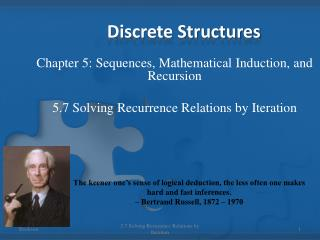Download PresentationDiscrete Structures

# Discrete Structures - PowerPoint PPT PresentationDownload Presentation## Discrete Structures

- - - - - - - - - - - - - - - - - - - - - - - - - - - E N D - - - - - - - - - - - - - - - - - - - - - - - - - - -
##### Presentation Transcript

1. Discrete Structures Chapter 5: Sequences, Mathematical Induction, and Recursion 5.7 Solving Recurrence Relations by Iteration The keener one’s sense of logical deduction, the less often one makes hard and fast inferences. – Bertrand Russell, 1872 – 1970 5.7 Solving Recurrence Relations by Iteration

2. Method of Iteration • The most basic method for finding an explicit formula for a recursively defined sequence is iteration. It works as follows: Given a sequence a0, a1, a2, … defined by a recurrence relation and initial conditions, you start from the initial conditions and calculate successive terms of the sequence until you see a pattern developing. At that point, you create an explicit formula. 5.7 Solving Recurrence Relations by Iteration

3. Example – pg. 315 #4 • Use iteration to guess an explicit formula for the sequence. Use formulas from Section 5.2 to simplify your answers whenever possible. Then use mathematical induction to verify the correctness of the formula you obtained. 5.7 Solving Recurrence Relations by Iteration

4. Example – pg. 315 #15 • Use iteration to guess an explicit formula for the sequence. Use formulas from Section 5.2 to simplify your answers whenever possible. Then use mathematical induction to verify the correctness of the formula you obtained. 5.7 Solving Recurrence Relations by Iteration

5. Definition • Geometric Sequence A sequence a0, a1, a2, … is called a geometric sequenceiff there is a constant rs.t. It follows that, 5.7 Solving Recurrence Relations by Iteration

6. Definition • Arithmetic Sequence A sequence a0, a1, a2, … is called an arithmetic sequenceiff there is a constant ds.t. It follows that, 5.7 Solving Recurrence Relations by Iteration

7. Example – pg. 315 # 20 A runner targets herself to improve her time on a certain course by 3 seconds a day. If on day 0 she runs the course in 3 minutes, how fast must she run it on day 14 to stay on target? 5.7 Solving Recurrence Relations by Iteration

8. Example – pg. 315 # 25 A certain computer algorithm executes twice as many operations when it is run with an input size k as when it is run with an input size k – 1 (where k is an integer greater than 1). When the algorithm is run with an input size of 1, it executes seven operations. How many operations does it execute when it is run with an input of size 25? 5.7 Solving Recurrence Relations by Iteration